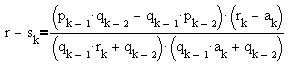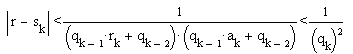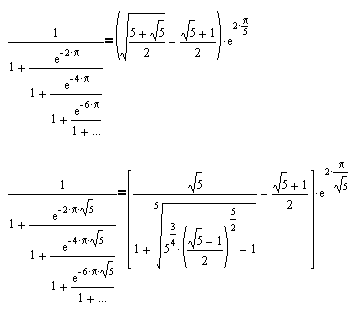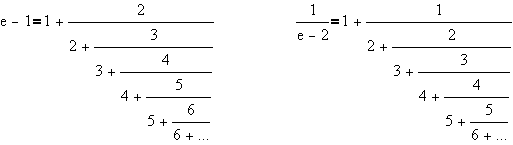The word 'fraction' derives from the Latin fractio - to break. However, there are continuous fractions.Well, not quite. The accepted terminology is continued fraction. However, one of the Russian terms for what I want to discuss on this page is indeed continuous fraction. Continued fraction are extremely important in the theory of rational approximation. They also provide a simple way to construct a variety of transcendental numbers. Here's the definition.

In general, continued fraction is an expression in the formwhere ai and bi may be any kind of numbers, variables, or functions. With all bi = 1 we have simple continued fractions.We shall only be concerned with the latter variety. A more convenient notation is r = [a0,a1,a2,a3,...]. To end with notations, if a0 is an integer, most often it's separated from the rest of the coefficients with a semicolon: r = [a0;a1,a2,a3,...]. In what follows I shall assume that all ai are integers and, for i > 0, ai > 0.

To make the terminology and notations more intuitive let's apply Euclid's algorithm to finding the greatest common divisor of, say, 1387 and 3796. Begin by dividing the smaller number 1387 into the larger one (3796) and keeping track of the remainder.

3796 = 1387·2 + 1022

which also can be written as

 3796/1387 = 2 + 1022/1387 = 2 + 1/(1387/1022).

The key step of the algorithm is exactly this: "Keep dividing the smaller number 1387 into the larger one." Thus, let's continue

 1387/1022 = 1 + 365/1022 = 1 + 1/(1022/365).

In other words,

 3796/1387 = 2 + 1022/1387 = 2 + 1/(1 + 1/(1022/365)).

Further

1022 = 365·2 + 292
365 = 292·1 + 73
292 = 73·4

Omitting parentheses (as is customary), we may write

 3796/1387 = 2 + 1022/1387 = 2+1/1+ 1/(1022/365) = 2+1/1+1/2+ 1/(365/292) = 2+1/1+1/2+1/1+1/4.

Finally, 3796/1387 = [2;1,2,1,4]. The above explains why the terms ai are usually called quotients. Also, it shows that every rational number can be associated with a finite continued fraction. On the other hand, given a finite fraction [a0;a1,a2,a3,...,an], we may as well carry out the arithmetic operations in the reverse order. Which will ultimately lead to a rational number r. Since, obviously, [a0;a1,a2,a3,...,an,1] = [a0;a1,a2,a3,...,an+1], let's agree to exclude the finite fractions with the last quotient equal to 1. With this convention, the correspondence between rational numbers and finite continued fractions becomes 1-1.

Irrational numbers can also be uniquely associated with (simple) continued fractions. Take an irrational r and denote a0 = [r], where [] is the floor function. Next write r = a0 + 1/r1, where r1 = 1/(r - a0). Continue in this fashion: a1 = [r1] and r2 = 1/(r1 - a1), and more generally

ak = [rk]
rk = 1/(rk-1 - ak-1),

where k > 1 (with the convention that r0 = r.) We can write

r = [a0;r1] = [a0;a1,r2] = [a0;a1,a2,r3] ...

Quite naturally the terms ri are called remainders. Note that, since, for an irrational r, 1 > r - [r] > 0, r1 = 1/(r - [r]) is also irrational and r1>1. The same is true for k > 1: all rk are irrational and rk > 1. Therefore the process never stops and leads to a uniquely defined infinite continued fraction for which ak > 0 for k > 0. Note that with thus defined correspondence r1 = [a1,r2] = [a1,a2,r3] = ..., r2 = [a2,r3] = ..., and so on.

As we see, the association between real numbers and continued fractions is defined recursively. It will come then as no surprise that many of the features of the continued fractions are expressed in recursive terms. For example, for a continued fraction (either finite or infinite) one defines a family of finite segments sk = [a0;a1,a2,...,ak], each sk being a rational number: sk= pk/qk with qk > 0. Then we have the following fundamental theorem that can be proved by induction.

## Theorem 1

For all k ≥ 2,

 (1) pk = akpk-1 + pk-2 (2) qk = akqk-1 + qk-2

Set p-1 = 1 and q-1 = 0. First subtracting (2) multiplied by pk-1 from (1) multiplied by qk-1 and then subtracting (2) multiplied by pk-2 from (1) multiplied by qk-2 we further get

## Theorem 2

For all k ≥ 0,

 (3) qkpk-1 - pkqk-1 = (-1)k (4) qkpk-2 - pkqk-2 = (-1)k-1ak

(3) and (4) can be rewritten as

 (3') sk - sk-1 = (-1)k-1/(qkqk-1) (4') sk - sk-2 = (-1)kak/(qkqk-2)It's about time I start drawing some conclusions.

1. From (2), qi grows with i.
2. All fractions sk with k >1 are irreducible. This follows from (3); for a common factor of pk and qk would divide 1.
3. From (4'), if k is even, sk is an increasing sequence. For k odd, sk decreases.
4. From (3'), if k is even, sk < sk-1. If k is odd, the reverse inequality holds.
5. Combining 3. and 4. we get

s0 < s2 < s4 < ... < s2n < ... < s2n-1 < ... < s5 < s3 < s1

6. Therefore, sk converges both for even and odd indices. From 1. and (3') the limits coincide. Thus, sk is a convergent sequence. It's a gratifying fact that the sequence converges to r. For this reason the fractions sk are known as Convergents. Let's prove this. As we know,

r = [a0;a1,...,ak-1,rk]

Therefore,

 (5) r = (pk-1rk + pk-2)/(qk-1rk + qk-2), k > 1.

On the other hand, obviously

 (6) sk = (pk-1ak + pk-2)/(qk-1ak + qk-2), k>1

From (5) and (6)which implieswhich, in view of 1., not only proves that sk converges to r but also gives an estimate for the rate of convergence. Note that this is the same inequality we once proved using the Pigeonhole principle. The new proof supplies a constructive way to approximate an irrational number.## Examples

1. r = [1;1,1,1,...] = 1 + 1/r. Therefore, r is the positive root of r 2 = r+1. Thus the golden ratio (1 + 5)/2 has the simplest continued fraction representation possible.
2. Similarly, you can find [2;2,2,2,...] and, in general, [n;n,n,...].
3. r = [1;2,2,2,...] can be found once you know [2;2,2,...]. But there is another way. r-1 = [0;2,2,2,...] whereas r+1 = [2;2,2,2,...]. Therefore (r - 1)(r + 1) = 1. Or r = 2.
4. r = [1;1,2,1,2,1,2,...]. Similar to the above, r+1 = [2;1,2,1,2,...] whereas r-1 = [0;1,2,1,2,...]. Let r1 = [1;2,1,2,...]. Then (r+1) = [2;r1] = 2·r1. On the other hand, (r-1) = [0; r1] = 1/r1. Thus we get (r+1)(r-1) = 2. Finally, r = 3.

All of the above fractions are periodic in the sense we apply to decimal fractions. Le comte J. L. Lagrange (1736-1813) proved that this is a characteristic property of roots of quadratic polynomials.

5. The next two examples are due to S. Ramanujan, one of the greatest mathematical geniuses. Being a poor uneducated clerk, in 1913 Ramanujan sent a letter to G. H. Hardy who was by this time a world famous mathematician. He was accustomed to receiving letters from cranks. So he was bored more than anything else. Then it dawned on him that "They must be true because, if they were not true, no one would have had the imagination to invent them." The examples below were among 120 theorems from the Ramanujan's letter and are specifically referred to in the above passage.6. The following couple belongs to L. Euler